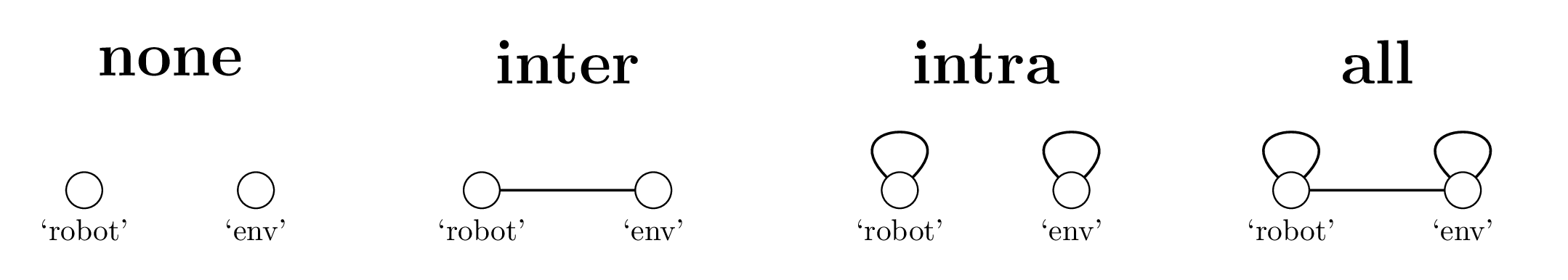# Collision Matrix¶

By default, collisions are turned off in pyrosim. To turn on collisions between rigid bodies they must be assigned collision groups and a collision matrix defining the interactions between those groups must be sent.

## Collision Groups¶

A collision group is a collection of bodies which all collide (or don’t) the same way with other collision groups. For example, if you do not want a robot to collide with its body parts, but want it to collide with objects in the environment, then all the bodies in the robot would be in one collision group and all the bodies in the environment would be in another.

When sending a body, you can assign a collision group name to the body by setting the collision_group parameter when the body is sent. The default name is ‘default’. After you have sent all the bodies you want in the simulation you use the name tag to define collisions

## Defining Collisions Between Groups¶

If we imagine each collision group as a node on a network, then it is easy to see the collision matrix as an adjacency matrix for this network where there is an edge between two groups if they collide and none if they do not. To go back to our robot/environment example above, we would want a connection between the ‘robot’ and ‘env’ collision groups and a loop connection on the ‘env’ node. Loops specify intra-group collision while edges between two different nodes specify inter-group collision.

If you do not want to specify every collision between every group you can use the predefined collision matrices provided by pyrosim. By using the sim.create_collision_matrix(collision_type) command you can choose from four different collision types: ‘none’, ‘inter’, ‘intra’, and ‘all’. They are depicted using the ‘env’ and ‘robot’ groups by the following figure:Regardless of how groups are defined and connected, the collisions between bodies which are attached by joints are disabled automatically. If they were enabled the simulation would most likely break.

## Double Pendulum Part IV¶

We have taken our double pendulum code and removed the sensors and turned the network it to a simple forward actuation about its connection in space. This simulation should show the pendulum actuate and hit a ball in the environment into the target blocks.

dp-collisions.py
import pyrosim
import random

# basically same code as previous examples with a few parameter tweaks
sim = pyrosim.Simulator(play_paused=True, debug=True, use_textures=True,
xyz=[-3.0, 2.0, 2.0], hpr=[-30.0, -15.0, 0.0],
eval_time=1000)

cyl_1 = sim.send_cylinder(x=-0.25, y=0.0, z=2.0,
r1=1, r2=0, r3=0,
collision_group='robot')
cyl_2 = sim.send_cylinder(x=-0.75, y=0.0, z=2.0,
r1=1, r2=0, r3=0,
collision_group='robot')

box = sim.send_box(x=-1.0, y=0.0, z=2.0,
width=0.5, length=0.5, height=0.5,
mass=10.0, collision_group='robot')

world_joint = sim.send_hinge_joint(first_body_id=pyrosim.Simulator.WORLD,
second_body_id=cyl_1,
x=0.0, y=0.0, z=2.0,
n1=0, n2=1, n3=0,
position_control=False,
torque=1000, speed=3)

hinge_joint = sim.send_hinge_joint(first_body_id=cyl_1,
second_body_id=cyl_2,
x=-0.5, y=0.0, z=2.0,
n1=0, n2=1, n3=0,
position_control=False,
torque=1000, speed=3)

slider_joint = sim.send_slider_joint(first_body_id=cyl_2,
second_body_id=box,
x=1.0, y=0.0, z=0.0,
lo=-0.5, hi=0.5)
# --------- New Code ----------------------------
# ball to kick
ball = sim.send_sphere(x=1.25, y=0, z=4, radius=.3,
r=.5, g=.5, b=.9,
collision_group='env')
# wall stack
SIZE = 1.0
for i in range(3):
color = random.random()
sim.send_box(x=3, y=0, z=(i + SIZE / 2.0) * SIZE,
width=SIZE, height=SIZE, length=SIZE,
mass=.2, r=.1, g=color, b=color,
collision_group='env')

# network is designed to just spin around the 'main' joint
bias_neuron = sim.send_bias_neuron()
motor_neuron = sim.send_motor_neuron(world_joint)
sim.send_synapse(bias_neuron, motor_neuron, -10.0)

pendulum = sim.get_group_id('robot')
environment = sim.get_group_id('env')

# here we can assign collisions directly
# we can also make a predefined collision matrix
# using sim.create_collision_matrix(collision_type)
# where collision_type = 'all','none','inter' or 'intra'.
sim.assign_collision('robot', 'env')
sim.assign_collision('env', 'env')

sim.start()
results = sim.wait_to_finish()